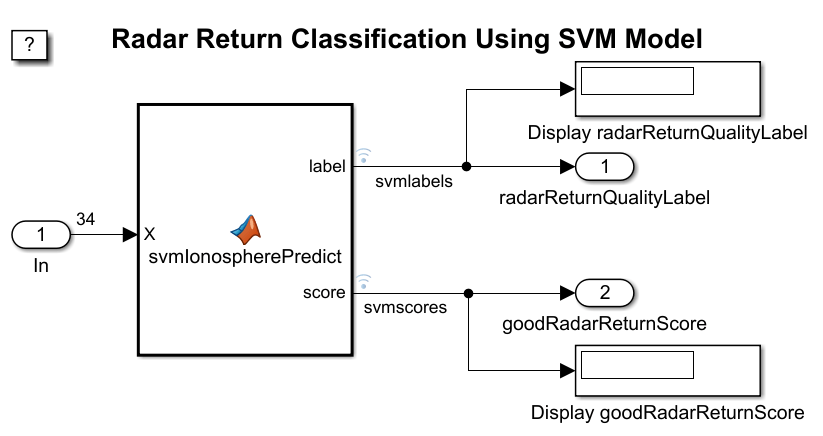Documentación

Esta página aún no se ha traducido para esta versión. Puede ver la versión más reciente de esta página en inglés.

## Predict Class Labels Using MATLAB Function Block

This example shows how to add a MATLAB Function block to a Simulink® for label prediction. The MATLAB Function block accepts streaming data, and predicts the label and classification score using a trained, support vector machine (SVM) classification model. For details on using the MATLAB Function block, see Create Custom Functionality Using MATLAB Function Block (Simulink).

The `ionosphere` data set, which is included in the Statistics and Machine Learning Toolbox™, contains radar-return qualities (`Y`) and predictor data (`X`). Radar returns are either of good quality (`'g'`) or of bad quality (`'b'`).

Load the `ionosphere` data set. Determine the sample size.

```load ionosphere n = numel(Y) ```
```n = 351 ```

The MATLAB Function block cannot return cell arrays. So, convert the response variable to a logical vector whose elements are `1` if the radar returns are good, and `0` otherwise.

```Y = strcmp(Y,'g'); ```

Suppose that the radar returns are detected in sequence, and you have the first 300 observations, but you have not received the last 51 yet. Partition the data into present and future samples.

```prsntX = X(1:300,:); prsntY = Y(1:300); ftrX = X(301:end,:); ftrY = Y(301:end); ```

Train an SVM model using all, presently available data. Specify predictor data standardization.

```Mdl = fitcsvm(prsntX,prsntY,'Standardize',true); ```

`Mdl` is a `ClassificationSVM` model. At the command line, you can use `Mdl` to make predictions for new observations. However, you cannot use `Mdl` as an input argument in a function meant for code generation.

Prepare `Mdl` to be loaded within the function using `saveCompactModel`.

```saveCompactModel(Mdl,'SVMIonosphere'); ```

`saveCompactModel` compacts `Mdl`, and then saves it in the MAT-file `SVMIonosphere.mat`.

Define a function named `svmIonospherePredict.m` that predicts whether a radar return is of good quality. The function should:

• Include the code generation directive `%#codegen` somewhere in the function.

• Accept radar-return predictor data. The data must be commensurate with `X` except for the number of rows.

• Load `SVMIonosphere.mat` using `loadCompactModel`.

• Return predicted labels and classification scores for predicting the quality of the radar return as good (that is, the positive-class score).

```function [label,score] = svmIonospherePredict(X) %#codegen %svmIonospherePredict Predict radar-return quality using SVM model % svmIonospherePredict predicts labels and estimates classification % scores of the radar returns in the numeric matrix of preditor data X % using the compact SVM model in the file SVMIonosphere.mat. Rows of X % correspond to observations and columns to predictor variables. label % is the predicted label and score is the confidence measure for % classifying the radar-return quality as good. % % Copyright 2016 The MathWorks Inc. Mdl = loadCompactModel('SVMIonosphere'); [label,bothscores] = predict(Mdl,X); score = bothscores(:,2); end ```

`svmIonospherePredict.m` is located in your `mlr/help/toolbox/stats/examples` folder, where `mlr` is the value of `matlabroot`.

Load the Simulink® model `slexSVMIonospherePredictExample.slx`.

```SimMdlName = 'slexSVMIonospherePredictExample'; open_system(SimMdlName); ```The figure displays the Simulink® model. When the input node detects a radar return, it directs that observation into the MATLAB Function block that dispatches to `svmIonospherePredict.m`. After predicting the label and score, the model returns these values to the workspace and displays the values within the model one at a time. When you load `slexSVMIonospherePredictExample.slx`, MATLAB® also loads the data set that it requires called `radarReturnInput`. However, this example shows how to construct the required data set.

The model expects to receive input data as a structure array called `radarReturnInput` containing these fields:

• `time` - The points in time at which the observations enter the model. In the example, the duration includes the integers from 0 though 50. The orientation must correspond to the observations in the predictor data. So, for this example, `time` must be a column vector.

• `signals` - A 1-by-1 structure array describing the input data, and containing the fields `values` and `dimensions`. `values` is a matrix of predictor data. `dimensions` is the number of predictor variables.

Create an appropriate structure array for future radar returns.

```radarReturnInput.time = (0:50)'; radarReturnInput.signals(1).values = ftrX; radarReturnInput.signals(1).dimensions = size(ftrX,2); ```

You can change the name from `radarReturnInput`, and then specify the new name in the model. However, Simulink® expects the structure array to contain the described field names.

Simulate the model using the data held out of training, that is, the data in `radarReturnInput`.

```sim(SimMdlName); ```The figure shows the model after it processes all observations in `radarReturnInput` one at a time. The predicted label of `X(351,:)` is `1` and its positive-class score is `1.431`. The variables `tout`, `yout`, and `svmlogsout` appear in the workspace. `yout` and `svmlogsout` are `SimulinkData.Dataset` objects containing the predicted labels and scores. For more details, see Data Format for Logged Simulation Data (Simulink).

Extract the simulation data from the simulation log.

```labelsSL = svmlogsout.getElement(1).Values.Data; scoresSL = svmlogsout.getElement(2).Values.Data; ```

`labelsSL` is a 51-by-1 numeric vector of predicted labels. `labelsSL(j)` = `1` means that the SVM model predicts that radar return `j` in the future sample is of good quality, and `0` means otherwise. `scoresSL` is a 51-by-1 numeric vector of positive-class scores, that is, signed distances from the decision boundary. Positive scores correspond to predicted labels of `1`, and negative scores correspond to predicted labels of `0`.

Predict labels and positive-class scores at the command line using `predict`.

```[labelCMD,scoresCMD] = predict(Mdl,ftrX); scoresCMD = scoresCMD(:,2); ```

`labelCMD` and `scoresCMD` are commensurate with `labelsSL` and `scoresSL`.

Compare the future-sample, positive-class scores returned by `slexSVMIonospherePredictExample` to those returned by calling `predict` at the command line.

```err = sum((scoresCMD - scoresSL).^2); err < eps ```
```ans = logical 1 ```

The sum of squared deviations between the sets of scores is negligible.

If you also have a Simulink® Coder™ license, then you can generate C code from `slexSVMIonospherePredictExample.slx` in Simulink® or from the command line using `rtwbuild`. For more details, see Generate C Code for a Model (Simulink Coder).

Download ebook